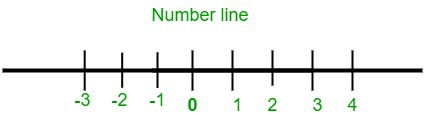# Is there a smallest number and a largest number?

• Last Updated : 12 Oct, 2021

The mathematical figures or digits used in various mathematical operations like addition, subtraction, division, multiplication, simplification, etc. are known as numbers or numerals. These numeral values are used in our day-to-day calculations as well as in financial, professional, and social fields for precise evaluations.

Numerals are the mathematical digits used for measuring, counting and other calculations.

Attention reader! All those who say programming isn't for kids, just haven't met the right mentors yet. Join the  Demo Class for First Step to Coding Coursespecifically designed for students of class 8 to 12.

The students will get to learn more about the world of programming in these free classes which will definitely help them in making a wise career choice in the future.

Number system is a method of representing numbers in the form of digits, symbols or words.

There are various types of numbers based on their numbering systems. The digit, place value, and the base of the number determine the value of the number. Some examples of numbers are integers, natural numbers, whole numbers, complex numbers, etc.

### Number system

Number system is a method of expressing standard numbers in the form of digits or words. It is an elementary system of representation mostly used in algebra and arithmetic. There are various methods of numbering under different number systems. The types of number systems are enlisted below:

• Binary number system (Base – 2)
• Octal number system (Base – 8)
• Decimal number system (Base – 10)
• Hexadecimal number system (Base – 16)

### Types of Numbers

In mathematics, several types of numbers are included. They are explained below:

• Natural number: Natural numbers are the positive counting numbers excluding zero. The numbers count from 1 to positive infinity. The set is represented by ‘N’ and consists of N={1,2,3,4,5,6,………………….}
• Whole number; Whole numbers are the positive counting numbers including zero. The numbers count from 0 to positive infinity. The set is represented by ‘W’. The set is represented by ‘W’ and consist of W={0,1,2,3,4,5,6,………………}
• Integers: Integers are the set of all positive and negative whole numbers counting from negative infinity to positive infinity. The set is represented by ‘Z’ and consists of Z={……………,-4,-3,-2,-1,0,1,2,3,,4,…………………….}
• Real number: Real numbers are the numeral values that don’t contain any imaginary value. it includes all the positive and negative integers along with fractions and finite decimals. The set is represented by ‘R’.
• Rational number: Rational numbers are any numbers that can be expressed in ratios. any number that can be represented in the form of p/q is a rational number.
• Irrational number: Irrational numbers are numbers that cannot be expressed as a ratio of one to another. It doesn’t qualify the p/q form of expression.

### Is there a smallest number and a largest number?

If we have a look at the set of integers we see that there is no such specific smallest or largest number in the set. The numbers put into the number line extends on both sides without any end up to positive and negative infinity.

Mathematically, the largest number is so large and unimaginable that it cannot be expressed in digits and the same concept goes for the smallest number too. Hence, these numbers are represented by a symbol named infinity (∞)### Similar Questions

Question 1. Which is the smallest and largest one-digit number?

According to mathematics the smallest one digit number is 1 and 9 is the largest one digit number.

Question 2. Which is the largest number known to humans?

The largest known number is googol. It is a unimaginably large number can be expressed in exponential form 10100.

Question 3. Do the numbers in the number line have an end?